Home    |    Teacher    |    Parents    |    Glossary    |    About UsEmail this page to a friendResources· Cool Tools · Formulas & Tables · References · Test Preparation · Study Tips · Wonders of MathSearchVolume Formulas
(Math | Geometry | Volume Formulas)
(pi =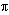= 3.141592...)

Be careful!! Units count. Use the same units for all measurements. Examples

cube = a 3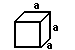rectangular prism = a b c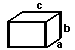irregular prism = b h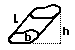cylinder = b h = pi r 2 h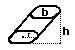pyramid = (1/3) b h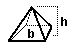cone = (1/3) b h = 1/3 pi r 2 h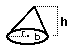sphere = (4/3) pi r 3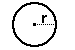ellipsoid = (4/3) pi r1 r2 r3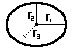Units

Volume is measured in "cubic" units. The volume of a figure is the number of cubes required to fill it completely, like blocks in a box.

Volume of a cube = side times side times side. Since each side of a square is the same, it can simply be the length of one side cubed.

If a square has one side of 4 inches, the volume would be 4 inches times 4 inches times 4 inches, or 64 cubic inches. (Cubic inches can also be written in3.)

Be sure to use the same units for all measurements. You cannot multiply feet times inches times yards, it doesn't make a perfectly cubed measurement.

The volume of a rectangular prism is the length on the side times the width times the height. If the width is 4 inches, the length is 1 foot and the height is 3 feet, what is the volume?

NOT CORRECT .... 4 times 1 times 3 = 12

CORRECT.... 4 inches is the same as 1/3 feet. Volume is 1/3 feet times 1 foot times 3 feet = 1 cubic foot (or 1 cu. ft., or 1 ft3).